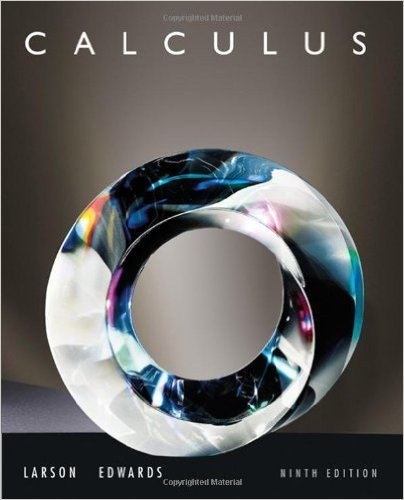×
×

# Solutions for Chapter 14.6: Triple Integrals and Applications## Full solutions for Calculus | 9th Edition

ISBN: 9780547167022Solutions for Chapter 14.6: Triple Integrals and Applications

Solutions for Chapter 14.6
4 5 0 240 Reviews
28
0
##### ISBN: 9780547167022

Chapter 14.6: Triple Integrals and Applications includes 76 full step-by-step solutions. Since 76 problems in chapter 14.6: Triple Integrals and Applications have been answered, more than 61684 students have viewed full step-by-step solutions from this chapter. Calculus was written by and is associated to the ISBN: 9780547167022. This expansive textbook survival guide covers the following chapters and their solutions. This textbook survival guide was created for the textbook: Calculus , edition: 9.

Key Calculus Terms and definitions covered in this textbook
• Absolute value of a real number

Denoted by |a|, represents the number a or the positive number -a if a < 0.

• Boxplot (or box-and-whisker plot)

A graph that displays a five-number summary

• Complex plane

A coordinate plane used to represent the complex numbers. The x-axis of the complex plane is called the real axis and the y-axis is the imaginary axis

• Convergence of a series

A series aqk=1 ak converges to a sum S if imn: q ank=1ak = S

• Coordinate(s) of a point

The number associated with a point on a number line, or the ordered pair associated with a point in the Cartesian coordinate plane, or the ordered triple associated with a point in the Cartesian three-dimensional space

• Graphical model

A visible representation of a numerical or algebraic model.

• kth term of a sequence

The kth expression in the sequence

• LRAM

A Riemann sum approximation of the area under a curve ƒ(x) from x = a to x = b using x1 as the left-hand endpoint of each subinterval

• Multiplicative identity for matrices

See Identity matrix

• Origin

The number zero on a number line, or the point where the x- and y-axes cross in the Cartesian coordinate system, or the point where the x-, y-, and z-axes cross in Cartesian three-dimensional space

• Parametric curve

The graph of parametric equations.

• Partial sums

See Sequence of partial sums.

• Projectile motion

The movement of an object that is subject only to the force of gravity

The graph in three dimensions of a seconddegree equation in three variables.

• Quantitative variable

A variable (in statistics) that takes on numerical values for a characteristic being measured.

• Reflection across the y-axis

x, y and (-x,y) are reflections of each other across the y-axis.

• Right angle

A 90° angle.

• Rigid transformation

A transformation that leaves the basic shape of a graph unchanged.

• Vertex of a cone

See Right circular cone.

• Vertical component

See Component form of a vector.

×